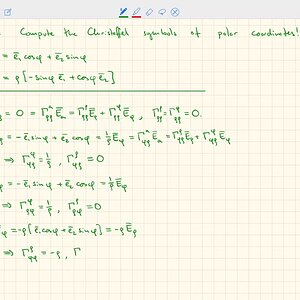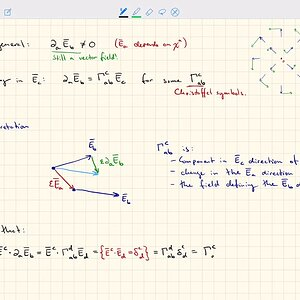# Christoffel symbols Definition and 18 Discussions

In mathematics and physics, the Christoffel symbols are an array of numbers describing a metric connection. The metric connection is a specialization of the affine connection to surfaces or other manifolds endowed with a metric, allowing distances to be measured on that surface. In differential geometry, an affine connection can be defined without reference to a metric, and many additional concepts follow: parallel transport, covariant derivatives, geodesics, etc. also do not require the concept of a metric. However, when a metric is available, these concepts can be directly tied to the "shape" of the manifold itself; that shape is determined by how the tangent space is attached to the cotangent space by the metric tensor. Abstractly, one would say that the manifold has an associated (orthonormal) frame bundle, with each "frame" being a possible choice of a coordinate frame. An invariant metric implies that the structure group of the frame bundle is the orthogonal group O(p, q). As a result, such a manifold is necessarily a (pseudo-)Riemannian manifold. The Christoffel symbols provide a concrete representation of the connection of (pseudo-)Riemannian geometry in terms of coordinates on the manifold. Additional concepts, such as parallel transport, geodesics, etc. can then be expressed in terms of Christoffel symbols.
In general, there are an infinite number of metric connections for a given metric tensor; however, there is a unique connection that is free of torsion, the Levi-Civita connection. It is common in physics and general relativity to work almost exclusively with the Levi-Civita connection, by working in coordinate frames (called holonomic coordinates) where the torsion vanishes. For example, in Euclidean spaces, the Christoffel symbols describe how the local coordinate bases change from point to point.
At each point of the underlying n-dimensional manifold, for any local coordinate system around that point, the Christoffel symbols are denoted Γijk for i, j, k = 1, 2, ..., n. Each entry of this n × n × n array is a real number. Under linear coordinate transformations on the manifold, the Christoffel symbols transform like the components of a tensor, but under general coordinate transformations (diffeomorphisms) they do not. Most of the algebraic properties of the Christoffel symbols follow from their relationship to the affine connection; only a few follow from the fact that the structure group is the orthogonal group O(m, n) (or the Lorentz group O(3, 1) for general relativity).
Christoffel symbols are used for performing practical calculations. For example, the Riemann curvature tensor can be expressed entirely in terms of the Christoffel symbols and their first partial derivatives. In general relativity, the connection plays the role of the gravitational force field with the corresponding gravitational potential being the metric tensor. When the coordinate system and the metric tensor share some symmetry, many of the Γijk are zero.
The Christoffel symbols are named for Elwin Bruno Christoffel (1829–1900).

View More On Wikipedia.org
1. ### B Christoffel Symbols of Hiscock Coordinates

The Hiscock coordinates read: $$d\tau=(1+\frac{v^2(1-f)}{1-v^2(1-f)^2})dt-\frac{v(1-f)}{1-v^2(1-f)^2}dx$$ ##dr=dx-vdt## Where ##f## is a function of ##r##. Now, in terms of calculating the christoffel symbol ##\Gamma^\tau_{\tau\tau}## of the new metric, where ##g_{\tau\tau}=v^2(1-f)^2-1## and...
2.### SH2372 General Relativity (3X): Christoffel symbols in polar coordinates

3.4. ### I Contracted Christoffel symbols in terms determinant(?) of metric

M. Blennow's book has problem 2.18: Show that the contracted Christoffel symbols ##\Gamma_{ab}^b## can be written in terms of a partial derivative of the logarithm of the square root of the metric tensor $$\Gamma_{ab}^b=\partial_a\ln{\sqrt g}$$I think that means square root of the determinant of...
5. ### I Covariant derivative of the Riemann tensor evaluated in Riemann normal

Hello everyone, in equation 3.86 of this online version of Carroll´s lecture notes on general relativity (https://ned.ipac.caltech.edu/level5/March01/Carroll3/Carroll3.html) the covariant derviative of the Riemann tensor is simply given by the partial derivative, the terms carrying the...

in video "Einstein Field Equation - for Beginner!" by "DrPhysicsA" on youtube, in 01:10:56, the christoffel symbol equation is written, then i see in "Physics Videos by Eugene Khutoryansky" video with title "Einstein's Field Equations of General Relativity Explained" in minute 05:02 on how the...

11. ### Dust in special relativity - conservation of particle number

Homework Statement My textbook states: Since the number of particles of dust is conserved we also have the conservation equation $$\nabla_\mu (\rho u^\mu)=0$$ Where ##\rho=nm=N/(\mathrm{d}x \cdot \mathrm{d}y \cdot \mathrm{d}z) m## is the mass per infinitesimal volume and ## (u^\mu) ## is...
12. A

### How Calculate Coriolis aceleration from Christoffel Symbols?

Homework Statement Hi, We are trying to calculate the Coriolis acceleration from the Cristoffel symbols in spherical coordinates for the flat space. I think this problem is interesting because, maybe it's a good way if we want to do the calculations with a computer. We start whit the...
13. ### I Is the Christoffel symbol orthogonal to the four-velocity?

Consider a force-free particle moving on a geodesic with four-velocity v^\nu. The formula for the four-acceleration in any coordinate system is \frac{dx^\mu}{d\tau} = - \Gamma^\mu_{\nu\lambda} v^\nu v^\lambda Since the four-acceleration on the left side is orthogonal to the four-velocity, this...
14. ### I Christoffel Symbol vs. Vector Potential

As far as I can tell, in GR, the Chirstoffel symbol in the expression of the Connection is analogous to the vector potential, A, in the definition of the Covariant Derivative. The Chirstoffel symbol compensates for changes in curvature and helps define what it means for a tensor to remain...
15. ### A Riemann tensor equation

The Riemann-Christoffel Tensor (##R^{k}_{\cdot n i j}##) is defined as: $$R^{k}_{\cdot n i j}= \frac{\delta \Gamma^{k}_{j n}}{\delta Z^{i}} - \frac{\delta \Gamma^{k}_{i n}}{\delta Z^{j}}+ \Gamma^{k}_{i l} \Gamma^{l}_{j n}- \Gamma^{k}_{j l} \Gamma^{l}_{i n}$$ My question is that it seems that...
16. ### I Exploring Curvature

Here's what I'm watching: At about 1:35:00 he leaves it to us to look at a parallel transport issue. Explicitly to caclculate ##D_s D_r T_m - D_r D_s T_m## And on the last term I'm having some difficulties, the second christoffel symbol. So we have ##D_s [ \partial_r T_m - \Gamma_{rm}^t T_t]##...
17. ### Trouble understanding ##g^{jk}\Gamma^{i}{}_{jk}##

Hi friends, I'm learning Riemannian geometry. I'm in trouble with understanding the meaning of ##g^{jk}\Gamma^{i}{}_{jk}##. I know it is a contracting relation on the Christoffel symbols and one can show that ##g^{jk}\Gamma^i{}_{jk}=\frac{-1}{\sqrt{g}}\partial_j(\sqrt{g}g^{ij})## using the...
18. ### Metric Tensor in R2

Hi, Want to know (i) what does Riemannian metric tensor and Christoffel symbols on R2 mean? (ii) How does metric tensor and Christoffel symbols look like on R2? It would be great with an example as I am new to this Differential Geometry.